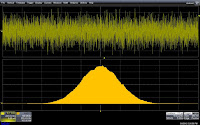## You need to test, we're here to help.

You need to test, we're here to help.

## 09 February 2015

### Using Histograms (Part I)Figure 1: Histograms of the period, width, andTIE show different distributions of time jitter
When we measure parameters of a waveform in a circuit or device, we rarely take a single measurement but rather a significant number of measurements. We want to see trends over time in the period, width, and time-interval error of a clock pulse, for example. Those parameters will have some nominal value, but there will typically be some random variation that we refer to as jitter.

Statistical analysis gives us a window to the view we want of this random variation in our DUT's behavior. A particularly handy tool in this vein is histograms, which many oscilloscopes (including most Teledyne LeCroy models) can generate as a means of visualizing and quantifying random processes (Figure 1). The histogram display shows the shape of the distribution of parameter values. Associated statistical parameters provide accurate, yet concise, measurements of that distribution. From this data, we can learn quite a bit about our signal.Figure 2: This histogram showsa random, or Gaussian,distribution of amplitude values

The distribution of measurement values shown in a histogram is related to the underlying process that generates the distribution. In Figure 2, we see an example of a random process that produces a Gaussian (or normal) distribution of amplitude values. The Gaussian distribution is a good indication that a random process is shaping variations in the measurement.Figure 3: The effect of passingGaussian noise throughan envelope detector
Now consider what happens when a Gaussian-distributed noise signal is applied to an envelope detector (Figure 3). In this case, the process involves full-wave rectification (an absolute-value function) and filtering (simulated in the oscilloscope using waveform math). Here, the distribution of amplitude values changes to a Rayleigh distribution. The amplitude values are no longer symmetric about the mean value (the effect of rectification). Knowledge of these effects allows us to calculate the expected noise power related to the input noise levels. Conversely, if the process was unknown, the measurement of the input and output distributions would help identify the operating process.

In Figure 4, the uniform distribution of delay is a characteristic of normal
operation in a timing synchronizer. This circuit synchronizes a random trigger event with a 400-MHz clock (2.5-ns period). If the input signal is independent of the system clock, then there is an equal probability of having any value of delay between the input and output over the range of one clock period.Figure 4: The uniform distributionof delay due to atiming synchronizer
In this example, note that the main distribution of the measured delay varies uniformly over a range of 2.5 ns as expected. However, a longer delay occasionally occurs, which shows up in the persistence display on Ch2 and as the secondary distribution in the histogram. By comparing the total populations of each section of the histogram, we find that the delayed event occurs 0.6% of the time. This highlights an advantage of the statistical study of measured data in that it quantifies rarely occurring events that might otherwise be overlooked.